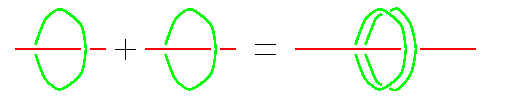© | << < ? > >> | Dror Bar-Natan: Talks:

# 1+1=2, The Hopf Link, and the Harish-Chandra-Duflo isomorphism

Dror Bar-Natan, Aarhus, November 1998.Computing 1+1=2 on an abacus.

One of the earliest demonstrations of the identity 1+1=2 was using the Chinese soroban (abacus). The proof is topological in nature: the number 1 is represented by a rod with a bead on it. The sum 1+1 would be taking two such rods and connecting them end to end, and the result is clearly topologically equivalent to the number 2, represented by a single rod with a double bead on it. In modern language, a "rod with a bead" is nothing but the Hopf link, with one of the components cut open, and the identity 1+1=2 becomes "the connected sum of two cut Hopf Links is equal to a single cut Hopf Link, with its uncut component doubled".

We apply the Stonehenge machinery to this identity, and get that two big sums of diagrams are equal (modulo some necessary relations). When a Lie algebra is given and these diagrams are interpreted as tensors in certain spaces associated with the Lie algebra, this equality becomes an easy proof of the multiplicativity property of the non-obvious Harish-Chandra-Duflo isomorphism.

The diagram equality we prove and use is the "Wheeling Conjecture" of Garoufalidis, Rozansky, D. Thurston and myself, first discovered in Aarhus some three years ago, and, independently, of Deligne. The 1+1=2 proof is due to D. Thurston and myself (Jerusalem, September 1998).